GSEB Solutions Class 12 Statistics Part 1 Chapter 2 Linear Correlation Ex 2.3

Gujarat Board Statistics Class 12 GSEB Solutions Part 1 Chapter 2 Linear Correlation Ex 2.3 Textbook Exercise Questions and Answers.

Gujarat Board Textbook Solutions Class 12 Statistics Part 1 Chapter 2 Linear Correlation Ex 2.3

Question 1.
Six companies are ranked by the two market analysts on the basis of their growth in the recent past:Find the rank correlation coefficient between the evaluation given by two analysts.
Here, n = 6; Rx = Ranks by Analyst 1; Ry = Ranks by Analyst 2.
The table for calculating rank correlation coefficient is prepared as follows :Rank correlation coefficient: r = 1 – \frac{6 \Sigma d^{2}}{n\left(n^{2}-1\right)}
Putting n = 6 and Σd2 =18 in the formula.
r = 1 – $$\frac{6(18)}{6\left(6^{2}-1\right)}$$
= 1 – $$\frac{108}{6(36-1)}$$
= 1 – $$\frac{108}{6 \times 35}$$
= 1 – $$\frac{108}{210}$$
= 1 – o.51
= 0.49
Hence, the rank correlation coefficient between the evaluation given by two analysts obtained is 0.49.Question 2.
An official has ranked nine villages of a sample on the basis of the work done in the area of ‘Swachhata Abhiyan’ and ‘Beti Bachavo Abhiyan’ by the villages. The ranks are given below: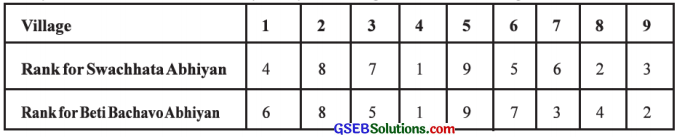Find the rank correlation coefficient between the performances of the villages in two Abhiyans.
Here, n = 9; Rx = Ranks for Swachhata Abhiyan and Ry = Ranks for Beti Bachavo Abhiyan
The table for calculating rank correlation coefficient is prepared as follows:Rank correlation coefficient: r = 1 – $$\frac{6 \Sigma d^{2}}{n\left(n^{2}-1\right)}$$
Putting n = 9 and Σd2 = 26 in the formula,
r = 1 – $$\frac{6(26)}{9\left(9^{2}-1\right)}$$
= 1 – $$\frac{156}{9(81-1)}$$
= 1 – $$\frac{156}{9 \times 80}$$
= 1 – $$\frac{156}{720}$$
= 1 – 0.22 = 0.78
Hence, the rank correlation coefficient between the performances of the villages in two Abhiyans obtained is 0.78.

Question 3.
The following information is obtained by a survey conducted by a town planning committee of a state :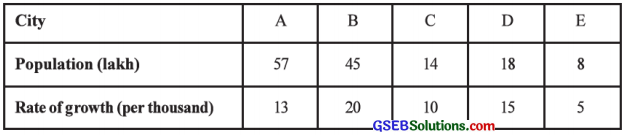Find the rank correlation coefficient between the population of the cities and the rate of growth of the population.
Here, n = 5;
Rx = Ranks for population (x) and
Ry = Ranks for rate of growth (y)
The table for calculating rank correlation coefficient is prepared as follows: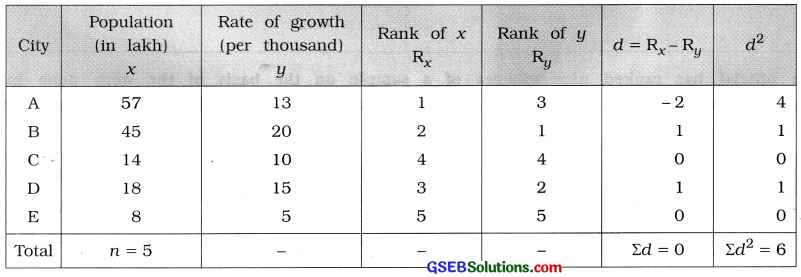Rank correlation coefficient: r = 1 – $$\frac{6 \Sigma d^{2}}{n\left(n^{2}-1\right)}$$
Putting n = 5 and Σd2 = 6 in the formula,
r = 1 – $$\frac{6(6)}{5\left(5^{2}-1\right)}$$
= 1 – $$\frac{36}{5(25-1)}$$
= 1 – $$\frac{36}{5 \times 24}$$
= 1 – $$\frac{36}{120}$$
= 1 – 0.3
= 0.7Question 4.
The following information is obtained by taking a sample of ten students from the students of a Science college: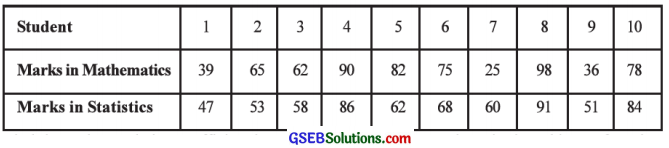Find the rank correlation coefficient between the ability of the students in the subjects of Mathematics and Statistics.
Here, n = 10; Rx = Ranks for marks in Mathematics (x) and Ry = Ranks for marks in Statistics (y).
The table for calculating rank correlation coefficient is prepared as follows: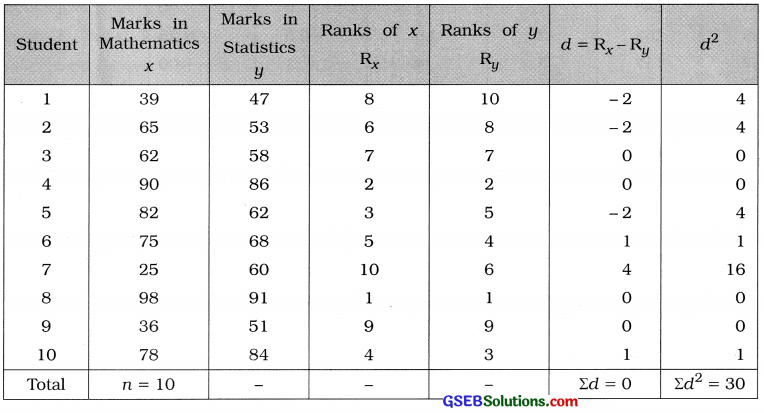Rank correlation coefficient: r = 1 – $$\frac{6 \Sigma d^{2}}{n\left(n^{2}-1\right)}$$
Putting n = 10 and Σd2 = 30 in the formula,
r = 1 – $$\frac{6(30)}{10\left(10^{2}-1\right)}$$
= 1 – $$\frac{180}{10(100-1)}$$
= 1 – $$\frac{180}{990}$$
= 1 – 0.18
= 0.82
Hence, the rank correlation coefficient between the ability of the students in the subjects of Mathematics and Statistics obtained is 0.82.

Question 5.
From the following information of heights of husband and wife, calculate the rank correlation coefficient between their heights:Here, n = 7; Rx = Ranks for height of husband (x) and Ry = Ranks for height of wife (y).
The table for calculating rank correlation coefficient is prepared as follows:Rank correlation coefficient:
Here, two observations 16.2 and 154 of the variable y are repeated twice. So correction factor CF is calculated as follows:Now, r = 1 – $$\frac{6\left(\Sigma d^{2}+\mathrm{CF}\right)}{n\left(n^{2}-1\right)}$$
Putting n = 7; Σd2 = 4 and CF = 1 in the formula,
r = 1 – $$\frac{6(4+1)}{7\left(7^{2}-1\right)}$$
= 1 – $$\frac{6(5)}{7(49-1)}$$
= 1 – $$\frac{30}{336}$$
= 1 – 0.09
= 0.91
Hence, the rank correlation coefficient between the heights of husband and wife is obtained 0.91.Question 6.
Two interviewers gave the following scores to the candidates on the basis of their performance in the interview. Find the rank correlation coefficient between the evaluation of two interviewers:Here, n = 8: Rx = Ranks for marks by first interviewer (x) and Ry = Ranks for marks by second interviewer (y).

The table for calculating rank correlation coefficient is prepared as follows: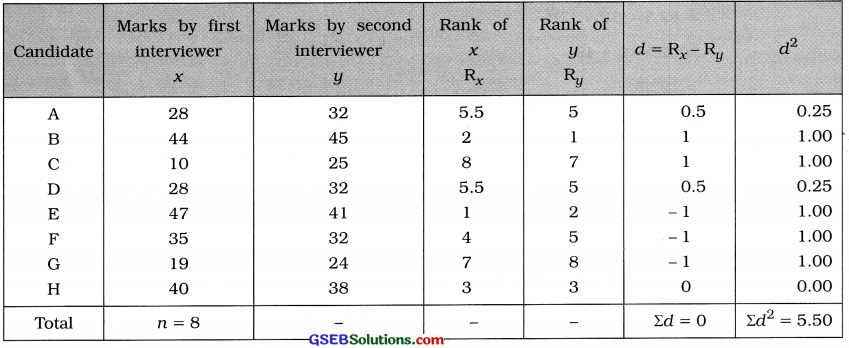Rank correlation coefficient:
Here, one observation 28 of x is repeated twice and one observation 32 of y is repeated thrice. We calculate CF as follows: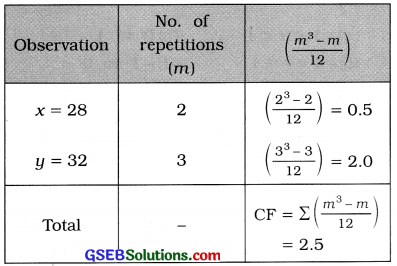Now, r = 1 – $$\frac{6\left(\Sigma d^{2}+\mathrm{CF}\right)}{n\left(n^{2}-1\right)}$$
Putting n = 8, Σd2 = 5.50 and CF = 2.5 in the formula,
r = 1 – $$\frac{6(5.50+2.50)}{8\left(8^{2}-1\right)}$$
= 1 – $$\frac{6(8)}{8(64-1)}$$
= 1 – $$\frac{48}{504}$$
= 1 – 0.10
= 0.90
Hence, the rank correlation coefficient between the evaluation of two interviewers obtained is 0.90.

Question 7.
Ten contestants are ranked in a beauty contest by two judges and the sum of squares of differences in their ranks is found to be 214. Find the rank correlation coefficient.
Here, n = 10; Σd2
Now, r = 1 – $$\frac{6\left(\Sigma d^{2}+\mathrm{CF}\right)}{n\left(n^{2}-1\right)}$$
Putting n = 10 and Σd2 = 214 in the formula,
r = 1- $$\frac{6(214)}{10\left(10^{2}-1\right)}$$
= 1 – $$\frac{1284}{10(100-1)}$$
= 1 – $$\frac{1284}{990}$$
= 1 – 1.30
= – 0.30
Hence, the rank correlation coefficient obtained is – 0.30.Question 8.
The coefficient of rank correlation of the marks obtained by 10 students in two particular subjects was found to be 0.5. Later on, it was found that one of the differences of the ranks of a student was 7 but it was taken as 3. Find the corrected value of the correlation coefficient.
Here, n = 8; r = 0.5
True difference = 7. False difference = 3
Now., r = 1 – $$\frac{6\left(\Sigma d^{2}+\mathrm{CF}\right)}{n\left(n^{2}-1\right)}$$ 2
Putting n = 10 and r = 0.5 in the formula.
0.5 = 1 – $$\frac{6 \Sigma d^{2}}{10\left(10^{2}-1\right)}$$
∴ $$\frac{6 \Sigma d^{2}}{10(100-1)}$$ = 1 – 0.5
∴ $$\frac{6 \Sigma d^{2}}{990}$$ = 0.5
∴ Σd2 = $$\frac{0.5 \times 990}{6}$$ = 82.5
Corrected Σd2 = 82.5 – (False d)2 + (True d)2
= 82.5 – (3)2 + (7)2
= 82.5 – 9 + 49
= 122.5
Corrected rank correlation coefficient:
r = 1 – $$\frac{6\left(\text { Corrected } \Sigma d^{2}\right)}{n\left(n^{2}-1\right)}$$
Putting n = 10 and corrected Σd2 = 122.5 in the formula.
r = 1 – $$\frac{6(122.5)}{10\left(10^{2}-1\right)}$$
= 1 – $$\frac{735}{990}$$
= 1 – 0.74
= 0.26
Hence, the corrected value of the coefficient of rank correlation obtained is 0.26.